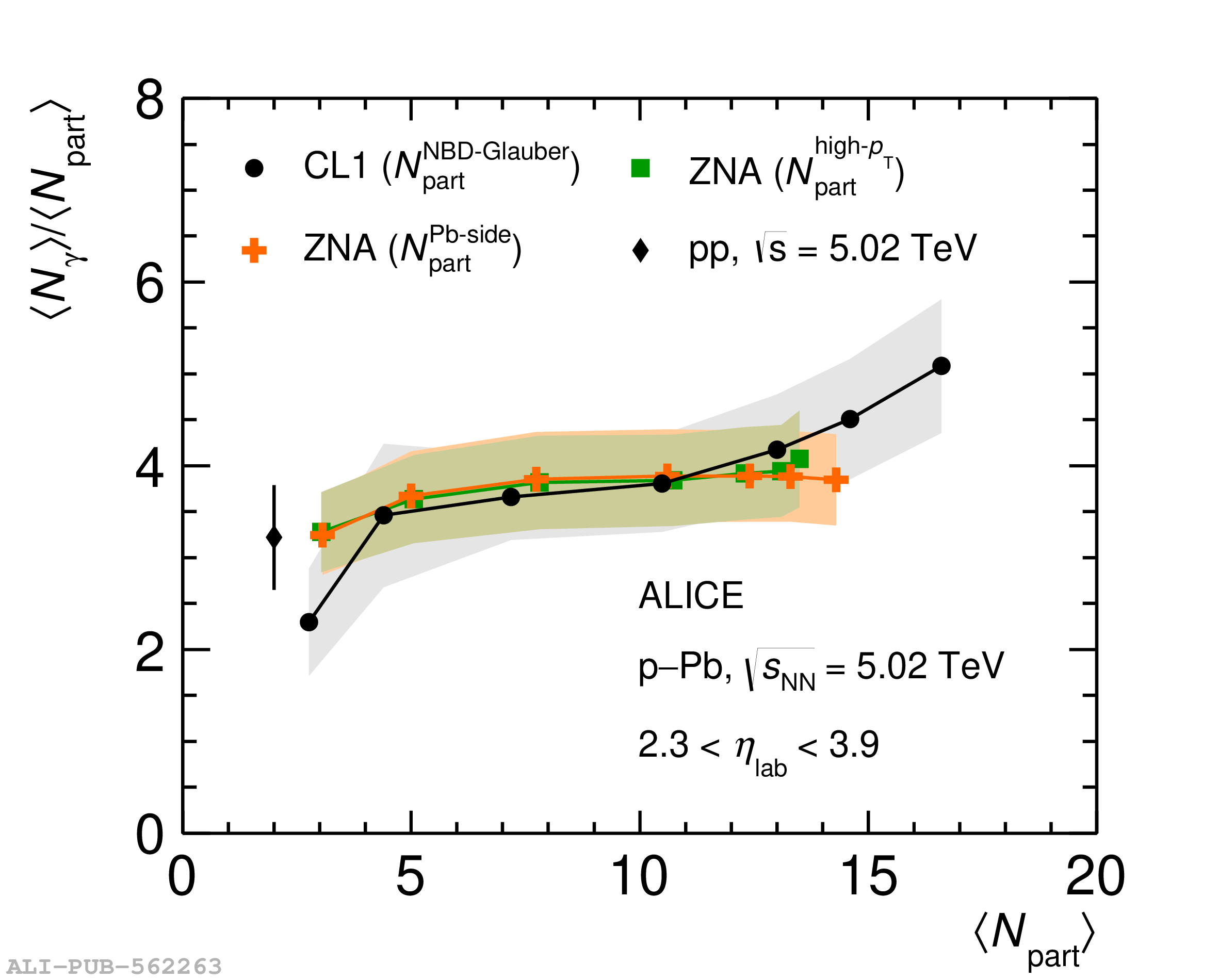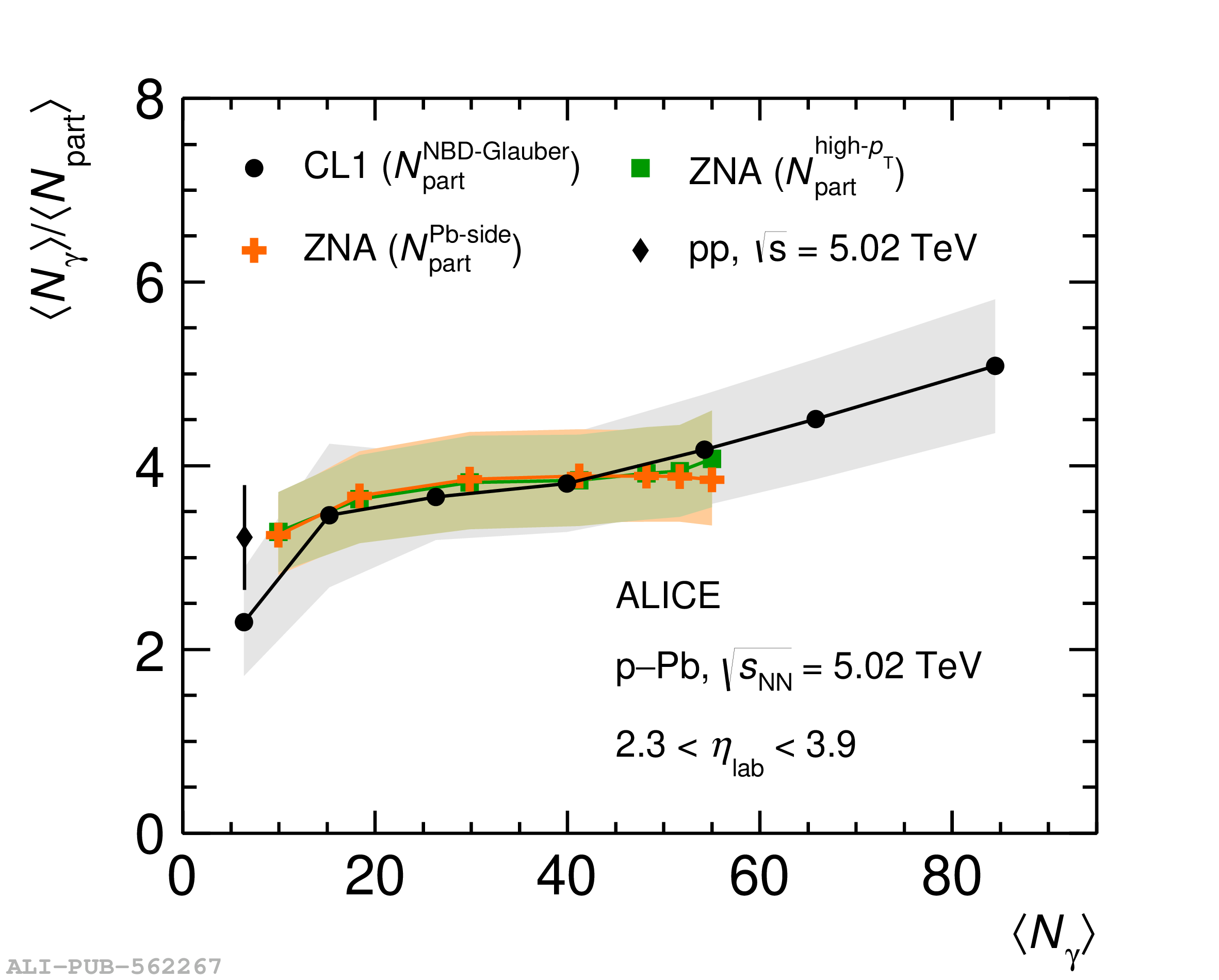# Figure 10

 (a) The average photon multiplicity $\langle N_{\rm \gamma} \rangle$ within 2.3 $< \eta_{\rm lab} <$ 3.9 as a function of the average number of participants for various centrality estimators in p$-$Pb collisions at $\sqrt{s_{\rm NN}}=5.02$ TeV. Values of $\langle N_{\rm \gamma} \rangle$/$\langle \Npart \rangle$ are shown as a function of $\langle \Npart \rangle$ (b) and $\langle N_{\rm \gamma} \rangle$ (c). The lines between the data points are to guide the eye. The data point for pp collisions at $\sqrt{s}=5.02$ TeV is also included for reference.